Next: Non-linear Perturbation Theories Up: Gravitational Instability Previous: Gravitational Instability

### Linear Perturbation Theory

In the following discussion of the evolution of the density contrast, we assume that Newtonian mechanics can be applied, an approximation which simplifies the study of the development of structures significantly compared to Einstein's theory of gravity. This assumption is well justified if we stay within the non-relativistic domain; i.e.and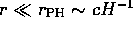(Hubble radius), where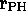is the size of the particle horizon and H is the Hubble parameter at time t.

The matter will be treated as an ideal fluid, which will enable us to make use of the following three equations: the conservation of mass'the Euler equation of motion,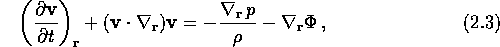and Poisson's equation for the gravitational potential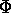,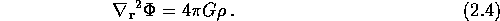The velocity fieldand the mass densityare both functions of the physical coordinatesand time t. The subscriptin the equations indicate that the term in question is to be calculated with respect to the proper coordinates. But, as in equation (2.1), we would like to use comoving coordinates. The relation between these two types of coordinates is as follows: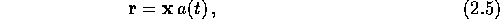where a(t) is the scale factor describing the scale of the Universe as it expands. The velocity field can then be written as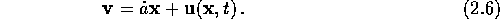The first term on the right hand side is recognized as the Hubble flow,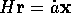, and the last term is the peculiar velocity relative to the general expansion,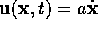. If we assume that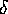and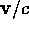have small values; i.e. much less then unity, we can linearize equations (2.2)-(2.4). This assumption is well justified in the early epochs of the Universe and on large-scales, where the mass distribution is approximately homogeneous. If we combine the linearized version of equations (2.2)-(2.4), we get the time evolution equation of the mass density contrast in linear perturbation theory: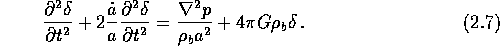This equation is local; i.e. it is only dependent on the conditions at one point in space. It was this equation Lif deduced.

Let us now take a look at some solutions of equation (2.7), assuming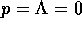, where p is pressure and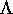is the cosmological constant. The easiest solution is for the Einstein-de Sitter model, where there is no space curvature (k=0). The Friedmann-equations then gives us the following time evolution of the scale parameter a: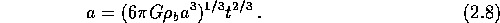If we insert this relation into equation (2.7), we get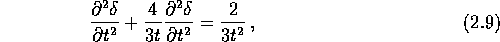a partial differential equation which has the solutionwhere A and B are constants. Based on this solution, Lif jumped to the conclusion that the gravitational instability theory could not be used to explain the formation of structures in the Universe; the growth would be too slow compared with the exponential growth in a non-expanding Universe, which was the leading model at the time.

Another solution whencan be found by rewriting the Friedmann-equations as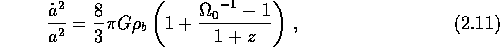where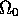is the density parameter and z is the cosmological redshift give by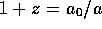(subscript ``0'' denotes the present value). At large redshifts; i.e.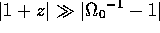, we get the Einstein-deSitter model, and equation (2.10) is valid for all models. If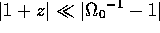we have an open model, and equation (2.11) will reduce to the Milne-model, where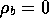and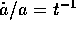. If we insert this into equation (2.7) we get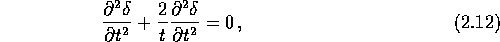which has the solutionThese two solutions in equation (2.10) and (2.13) tell us that the perturbations will, assuming amodel, initially grow, but later, after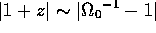, grow slower and finally end up with a constant amplitude.

So far we have ignored any pressure in the fluid, which at early epochs of the Universe is a bad assumption. If we introduce pressure, the time evolution equation or the mass density contrast will be coordinate dependent, which was not the case for p=0. If we simplify the picture by assuming an ideal gas, we can, with help of equation (2.1), deduce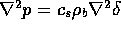, where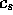is the speed of sound. This brings the density perturbation equation (2.7) toTo see the effect of the pressure term, we can write the density contrast as a Fourier series,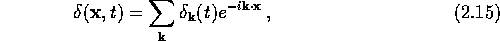where the proper wavelength is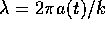. The coefficients in equation (2.15) is independent of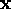, which gives us the following equation for each number vector:The source terms on the right-hand side vanishes at wavelengthwhich is often referred to as the Jeans length. This was the scale Jeans-02 deduced as being the maximum size a gas cloud could have without collapsing. What does equation (2.16) tell us? Let's take a look at two special cases: If; i.e. the wavelengths are long compared to the Jeans length, the pressure term in equation (2.14) becomes negligible, and we can apply the zero pressure solution. At wavelengths shorter then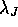, the density contrast oscillates as a sound wave, which means that a density perturbations can not grow if it's scale is less then the Jeans length. If we have perturbations with amplitude decreasing with scale, the ones with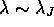will be the first to become non-linear.Next: Non-linear Perturbation Theories Up: Gravitational Instability Previous: Gravitational Instability

Trond Hjorteland
Mon Jul 5 02:59:28 MET DST 1999